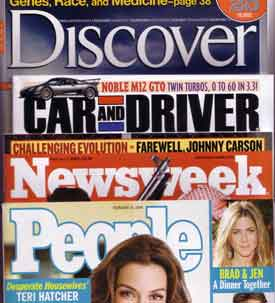WORKSHEET:ALGEBRA Worksheets Descargar PDF 1) Write a equation for each sentence: a. Three added to four times a number is equal to fifteen Solution b. One third of a number is equal to seven Solution c. The cost of x chocolate boxes, if each chocolate box costs 15 is 540Solution d. Seven subtracted from three times a number is equal to eight Solution e. How many chocolates will each boy get if x chocolates are to be shared equally among six boys? Solution f. The difference between a number and eleven is equal to five. Solution g. Half a number is equal to seventeen. Solution h. Twice a number plus two is equal to 18. Solution i. One third of a number minus four is equal to seventeen. Solution j. One quarter of a number plus five is equal to nine. Solution 2) For each sentence, decide which equation matches it. I) Five more than the double of a number is 25. a) 2n + 5 = 25 b) 2+5+n = 25 c) 2 + 5n = 25 d) 2  5n = 25 Solution II) The difference between 20 and twice a number is 8. a) 20  2n = 8 b) 20  8 = 2n c) 2·8  n = 20 d) 2n  8 = 20 Solution III) Carol spent 39 on n magazines. They cost 3 each. a) 39n = 3 b) n/3 = 39 c) 3/n = 39 d) 3n = 39Solution IV) One third of the number that comes after my number is equal to 12. a) x/3 = 12 b) (x  1)/3 = 12 c) (x + 1)/3 = 12 d) x/3 + 1 = 12 Solution